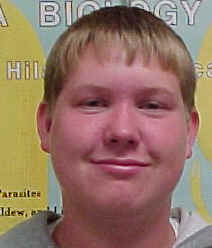# Bi Sample Metric Measure

 Metric Measurement LabIntroduction:

Every scientific experiment in some way involves measurement.  Scientists worldwide use the metric system to display the results of measurements.  This system simplifies calculations based on a decimal system (powers of ten), opposed to the confusing English system of measurement.  Less confusion and better communication between scientists around the world makes the metric system more efficient than the English system for use in experiments.  The useful prefixes of the metric system are also known as the International System of Units (SI).

Two measurements explored in this lab are mass and volume.  Mass is represented by grams, while volume is represented by milliliters (liquid) and cubic centimeters (solids with ruler measurement).  The purpose of this investigation is to get acquainted with and be accurate with the metric system.

Hypothesis:

By using a graduated cylinder, mass balance, and metric ruler, mass and volume can be found.

Materials:

The materials used in this experiment include a graduated cylinder, an eyedropper, and a beaker of water for Part A; 20 ml of water, a graduated cylinder, and three marbles for Part B; a metric ruler, mass balance, three marbles, and a graduated cylinder for Part C; a metric ruler for Part D; and a graduated cylinder, eyedropper, six labeled test tubes, and three 25ml beakers of colored water (one with red, one with blue, and one with yellow) for Part E.

Methods:

Part A:  Count you drops!

Fill a small graduated cylinder with 10 ml of water.  Count and record the number of drops it takes to raise the water to 11ml.  Leave the water in the graduated cylinder and count and record the number of drops it takes to raise the water to 12ml.  Leave the water in the graduated cylinder and count and record the number of drops it takes to raise the water to 13ml.  Calculate the average number of drops and round to the nearest tenth.

Part B:  Water Displacement

Add 20ml of water to a 100ml graduated cylinder.  Record this amount in the chart.  Add three marbles to the cylinder and measure and record the volume.  Find the difference between the two measurements and record it in the chart.  The difference between the two measurements will be the volume of the three marbles.

Part C:  Mass Mania

Check to see that the Pinter on the balance is pointing to zero.  If it is not, check to see that all the Riders (weights) are all the way to the left at the Zero mark.  Adjust the balance by turning the Adjustment Screw slowly until it points at zero.  Place the metric ruler on the pan and read and record the ruler’s mass.  After resetting the balance to zero, measure and record the mass of the empty 50ml graduated cylinder and then the three marbles.  Reset the balance to zero when all items have been massed.

Part D:  Volume by Formula

Use the formula Volume=length x width x height to find the volume of the box.  Measure to the nearest centimeter before calculating the answer.  If necessary, round the answer to two decimal places.

Part E:  Color Challenge

Obtain the following items from the teacher:  3 beakers with colored water-25ml of each color (red, blue, and yellow), 1 graduated cylinder (25ml to 50ml), 1 eyedropper, and 6 test tubes labeled A, B, C, D, E, and F.  Perform each of the following steps using accurate measurements.  Measure 17ml of red water from the beaker and po9ur it into test tube A.  Measure 21ml of yellow water from the beaker and pour it into test tube C.  Measure 22ml of blue water from the beaker and pour it into test tube E.  Measure 5ml of water from test tube A and pour it into test tube B.  Measure 6ml of water from test tube C and pour it into test tube D.  Measure 8ml of water from test tube E and pour it into test tube F.  Measure 5ml of water from test tube C and pour it into test tube B.  Measure 2ml of water from test tube A and pour it into test tube F.  Measure 4ml of water from test tube E and pour it into test tube D.  Record the results in the chart.

Results:

 # of drops to 11ml # of drops to 12ml # of drops to 13ml Average 24 26 25 25
1. Take a guess—how many drops of water will it take to equal 1 milliliter?  18 drops.
2. Based on your average, how close were you to your guess?  7 drops off of average.
3. Based on your average, how many drops would it take to make 1 liter?  25,000 drops.

Part B:  Water Displacement

 Volume of Water Before Adding Marbles (ml) Volume of Water After Adding Marbles (ml) Difference in Volume (ml) Volume of 3 marbles (ml) 20ml 25ml 5ml 5ml

Part C:  Mass Mania

 Mass of Metric Ruler (g) Mass of Empty 50ml graduated cylinder (g) Mass of 3 Marbles (g) 3.0g 31.5g 11.0g

Part D:  Volume by Formula

Volume= length x width x height

7.0 cm x 1.0 cm x 3.0 cm = 21.0 cubic centimeters

Part E:  Color Challenge

 Test Tube Color Final Volume (ml) A Red 10ml B Orange 10ml C Yellow 10ml D Green 10ml E Blue 10ml F Purple 10ml

Discussion and Conclusion: